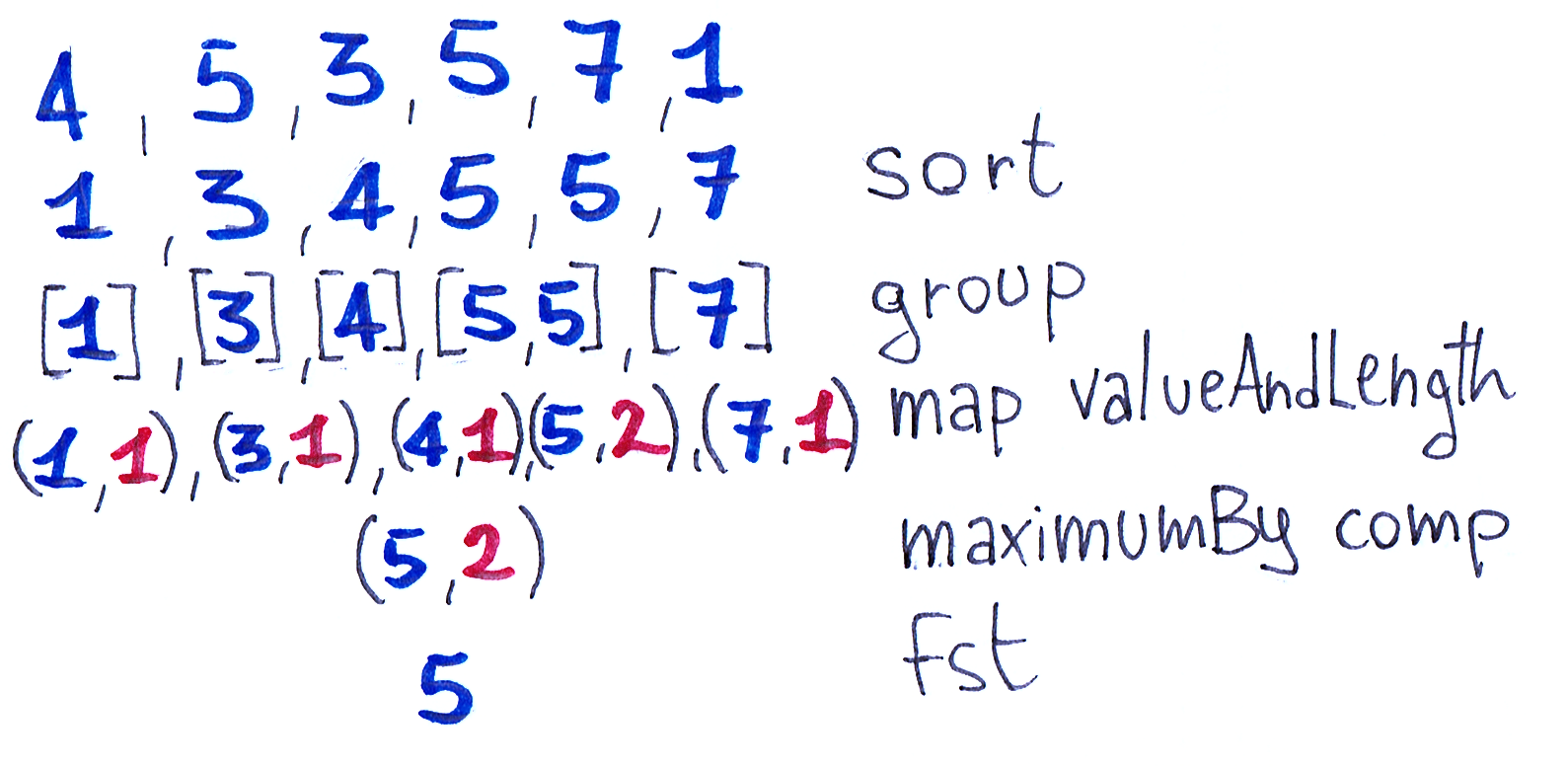# The Most Frequent

See the original problem on HackerRank.

You are given an array of N integers separated by spaces, all in one line.

Display the element which occurs most frequently. If multiple elements satisfy this criteria, display the numerically smallest one.

### Input Format

The first line contains the number of integers $$N$$.

The second line contains space separated integers $$xi$$ for which you need to find the most frequent one.

### Constraints

• $$10 \leq N \leq 1'000'000$$
• $$0 \leq xi \leq 100'000$$

### Output Format

One line containing the result.

### Example

Input:

 1 2  10 14470 11735 14216 99233 14470 4978 73429 38120 4978 67060 

Output:

 1  4978 

Explanation:

14470 and 4978 are the most frequent elements.

4978 is smaller so it is the answer.

## Solutions

### Frequency table

A C++ Solution based on the observation that the domain is quite small so we can use a frequency table:

 1 2 3 4 5 6  int elem; cin >> elem; array histogram{}; // we know i is within [0, 10^5] while (cin >> elem) histogram[elem]++; auto maxElem = std::max_element(begin(histogram), end(histogram)); cout << std::distance(begin(histogram), maxElem); 

Alternative C++ solution by Filippo Pinton

  1 2 3 4 5 6 7 8 9 10 11 12 13 14 15 16 17 18 19 20 21 22  int main() { int arr {}; int n, temp; int max = 0; int maxInt; std::cin >> n; for(int i = 0; i < n; i++) { std::cin >> temp; arr[temp] += 1; } for(int i = 0; i < 1000000; i++) { if(arr[i] > max) { max = arr[i]; maxInt = i; } } std::cout << maxInt << std::endl; return 0; } 

### Sort

We can solve this problem in a functional way, without allocating a frequency table. This is especially useful with bigger domains.

  1 2 3 4 5 6 7 8 9 10  import Data.List (group, maximumBy, sort) import Data.Ord (comparing) theMostFrequent :: [Int] -> Int theMostFrequent = fst . maximumBy comp . fmap valueAndLength . group . sort where comp = comparing snd <> comparing (negate . fst) valueAndLength xs = (head xs, length xs) main = interact $show . theMostFrequent . fmap read . tail . words  We first sort the list, then group by value, count groups items, and find the maximum comparing frequency and (negated) value.Ruby solution by Thomas Rossetto   1 2 3 4 5 6 7 8 9 10 11 12  s =$stdin.read splitted = s.split.drop(1).map(&:to_i) map = {} map = splitted.group_by(&:itself).transform_values!(&:size) if map.values.uniq.count == 1 puts map.keys.sort.first else map = map.sort_by {|key, value| [value, -key]} puts map.last end 
We've worked on this challenge in these gyms: modena  padua  milan